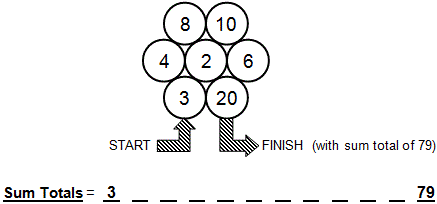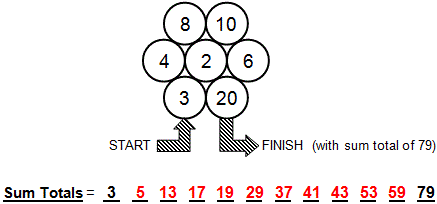Select PagePrintable version

In mathematics, a ‘Prime Number’ (also known as ‘Prime’) is defined as any ‘whole’ number that can be divided only by exactly two different, distinct factors (or divisors), 1 and the number itself; and must be greater than 1. By technical definition, 1 is not Prime because 1 = 1 x 1 and therefore the relationship between the number and its prime factors is no longer exclusive).  All ‘whole’ numbers (i.e., those that exclude fractions, percentages and decimals) can be factored or subdivided into one ‘unique’ set of Prime Factors that when multiplied together, equal the original number.  Primes can thus be considered the ‘basic building blocks’ of any whole number. The only ‘even’ number that is a Prime is 2.

Puzzle one

In the following Maths challenge, you travel from ‘START’ to ‘FINISH’ in no specific order or direction from one adjacent circle to another, keeping the accumulating sum total always equal to another ‘Prime’ number. Values in adjacent circles can be used more than once but ‘ping-ponging’ between circles (i.e., returning immediately to the circle that you have just left) is not allowed. The following challenge is to finish with a sum total of 79 (a Prime number).### SolutionPuzzle two

Twin Primes are a pair of Prime numbers that have a difference of 2.  How many pairs of twin primes are there less than 100?### Solution## Feedback

There are more than one way of doing these puzzles and may well be more than one answer.  Please let me and others know what alternatives you find by commenting below.  We also welcome general comments on the subject and any feedback you’d like to give.   If you have a question that need a response from me or you would like to contact me privately, please use the contact form.

## Get more puzzles!

If you’ve enjoyed doing the puzzles, consider ordering the book; 150+ of the best puzzles in a handy pocket sized format. Click here for full details.